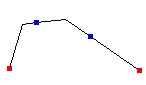Go to ET GeoWizards 12.x User Guide

Split Polyline Wizard

Go to ToolBox Implementation

Splits the features of a polyline layer.

Inputs:

• A polyline layer which features are to be split
• A method to be used for splitting
• In all vertices
• Equal segments
• Segments length - two options available
• Exact length + remainder - the polylines will be split using the exact length specified by the user. The last segment will have length equal to the remainder of the splitting. For example if a polyline with length = 60 meters is split using length of 25 meters, three segments with lengths 25, 25 and 10 will be created.
• Equal Length - the user specified length is adjusted in order all resulting segments to have equal length. For example if a polyline with length = 60 meters is split using length of 25 meters, two segments with lengths of 30 meters will be created.
• Number of vertices per feature
• Attribute update rules for each field

Outputs:

• A polyline feature class - new node created in each split point. The attributes will be distributed according the user specified attribute update rules.

Notes:

• Range attributes from string and numeric fields can be handled. The records that have range values containing non numeric characters will be copied to the resulting features.
• If the "Split selected features only" option is selected only the selected features from the input layer will be split.
• If the Segment length method is used
• all the segments will have the user specified length except for the last one
• if the assigned length is larger than the length of a specific polyline, the polyline will be copied to the output as is
• the splitting starts always from the start points (From Node) of the polylines

Examples:

 Before Split Split in all Vertices Split in equal segments Split with segment lengthCommand line syntax

ET_GPSplitPolylines<input_dataset> <out_feature_class> <NumberIntervals | NumberVertices | SegmentLength | EqualLength | Vertex> <split_tolerance>

Parameters

Expression Explanation
<input_dataset> A Polyline or Polygon feature class or feature layer
<out_feature_class> A String - the full name of the output feature class (A feature class with the same full name should not exist)
<NumberIntervals | NumberVertices | SegmentLength | EqualLength | Vertex> A String -  the split option to be used.
<split_tolerance>  Required. A Double representing depending on the option selected:
• "Vertex" - not used.
• "NumberIntervals" - the number of segments
• "SegmentLength" - the length of the segments
• "EqualLength" - the length of the segments
• "NumverVertices"- the number of vertices per segment
{Update_Rules_List} A String - a list of fields with their update rules.

Scripting syntax

ET_GPSplitPolylines(input_dataset, out_feature_class, split_option, split_tolerance, Update_Rules_List)

See the explanations above:
<> - required parameter
{} - optional parameter

Example Python script:

 import arcpyarcpy.ImportToolbox("C:/Program Files/ET SpatialTechniques/ET GeoWizards 11.0 for ArcGIS 10.1/ET GeoWizards.tbx")arcpy.gp.toolbox = "C:/Program Files/ET SpatialTechniques/ET GeoWizards 11.0 for ArcGIS 10.1/ET GeoWizards.tbx"input_dataset = "C:\\data\\streets.shp"result = "C:\\data\\fgdb_test.gdb\\split"arcpy.gp.ET_GPSplitPolylines (input_dataset, result, "SegmentLength",50, "Meters Proportion; Suburb Copy; L_F_ADD Range Address L_T_ADD ;L_T_ADD Range Address L_F_ADD")